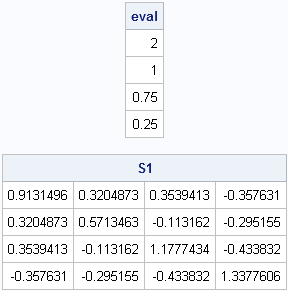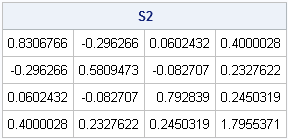In a previous post I showed how to implement Stewart's (1980) algorithm for generating random orthogonal matrices in SAS/IML software. By using the algorithm, it is easy to generate a random matrix that contains a specified set of eigenvalues.

If D = diag(λ1, ..., λp) is a diagonal matrix and Q is a pxp orthogonal matrix, then Q`DQ has eigenvalues λ1, ..., λp. Therefore if you choose Q at random, you obtain a random matrix with a given set of eigenvalues, as shown in the following statements:

```proc iml; load module=RndOrthog; /* load or define modules here */   start RndMatWithEigenval(lambda); n = ncol(lambda); /* assume lambda is row vector */ Q = RndOrthog(n); return( Q`*diag(lambda)*Q ); finish;   lambda = {2 1 0.75 0.25}; call randseed(1234); S1 = RndMatWithEigenval(lambda); /* eigenvalues are lambda */ eval = eigval(S1); print eval; print S1;```If you call the function a second time, you get a different matrix with the same eigenvalues:

```S2 = RndMatWithEigenval(lambda); /* eigenvalues are lambda */ print S2;```The ability to generate random matrices with specified eigenvalues is useful in simulation studies. Stewart's original motivation was to generate matrices with arbitrary condition number. The condition number of a matrix (in the two-norm) is the square root of of the ratio of the largest eigenvalue to the smallest eigenvalue. The condition number is important in numerical analysis because matrices with large condition numbers often lead to numerical instabilities in matrix computations. The algorithm implemented in this article provides a convenient way to generate matrices with an arbitrary condition number.

You can use these matrices in statistical simulations as well. In a future blog post, I will show how to transform a random matrix into a correlation matrix with the same set of eigenvalues. The correlation matrix can be used to generate multivariate normal data with a given correlation by using the Cholesky transformation technique.

Share